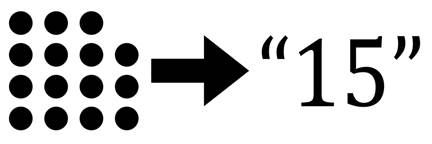# Exercise #31: Convert Integers To Strings

convertIntToStr(42)   '42'In Python, the values `42` and '42' are two different values: you can perform mathematics on the integer `42`, but the string '42' is the same as any other two-character text string. You can perform mathematical addition on integer values, but not on strings. And you can concatenate or replicate string values, but not integers. A common programming task is to obtain the string equivalent of a number. You can use the `str()` function to do this conversion but in this exercise you’ll recreate this function yourself.

Exercise Description

Write a `convertIntToStr()` function with an `integerNum` parameter. This function operates similarly to the `str()` function in that it returns a string form of the parameter. For example, `convertIntToStr(42)` should return the string `'42'`. The function doesn’t have to work for floating-point numbers with a decimal point, but it should work for negative integer values.

Avoid using Python’s `str()` function in your code, as that would do the conversion for you and defeat the purpose of this exercise. However, we use `str()` with assert statements to check that your `convertIntToStr()` function works the same as `str()` for all integers from `-10000` to `9999`:

for i in range(-10000, 10000):

assert convertIntToStr(i) == str(i)

Try to write a solution based on the information in this description. If you still have trouble solving this exercise, read the Solution Design and Special Cases and Gotchas sections for additional hints.

Prerequisite concepts: dictionaries, `while` loops, string concatenation, integer division

Solution Design

To create the string equivalent of the integer value, we’ll convert the integers to strings one digit at a time. Let’s create a variable named stringNum to hold the converted string. In a loop, the expression `integerNum % 10` evaluates to the digit in the one’s place of `integerNum`. Store this result in a variable named `onesPlaceDigit`.

Then we add the string equivalent of `onesPlaceDigit` to `stringNum` with a series of `if`-elif statements such as the following:

if onesPlaceDigit == 0:

stringNum = '0' + stringNum

elif onesPlaceDigit == 1:

stringNum = '1' + stringNum

elif onesPlaceDigit == 2:

stringNum = '2' + stringNum

elif onesPlaceDigit == 3:

stringNum = '3' + stringNum

elif onesPlaceDigit == 4:

stringNum = '4' + stringNum

elif onesPlaceDigit == 5:

stringNum = '5' + stringNum

elif onesPlaceDigit == 6:

stringNum = '6' + stringNum

elif onesPlaceDigit == 7:

stringNum = '7' + stringNum

elif onesPlaceDigit == 8:

stringNum = '8' + stringNum

elif onesPlaceDigit == 9:

stringNum = '9' + stringNum

However, this code is pretty long and tedious. A more concise and common approach is to create a dictionary that maps individual integer digits to their string equivalents, and then look up the value for the onesPlaceDigit key:

DIGITS_INT_TO_STR = {0: '0', 1: '1', 2: '2', 3: '3', 4: '4', 5: '5', 6: '6', 7: '7', 8: '8', 9: '9'}

stringNum = DIGITS_INT_TO_STR[onesPlaceDigit] + stringNum

After this, we can integer divide it by `10` to “remove” the digit in the one’s place of `integerNum`. For example, `41096 // 10` evaluates to 4109, effectively removing the `6` from the number and making the `9` the new digit in the one’s place to convert. Our loop can continue looping and converting digits until `integerNum` is `0`. For example, doing this to the integer 41096 would carry out the following operations:

·       41096 // 10 = 4109

·       4109 // 10 = 410

·       410 // 10 = 41

·       41 // 10 = 4

·       4 // 10 = 0

At this point the algorithm is finished and `stringNum` contains the string form.

Special Cases and Gotchas

At the start of the function, check if the `integerNum` parameter is `0` and, if so, immediately return the string `'0'`. Our algorithm is such that otherwise it will return the blank string `''` for 0, which is incorrect.

Now try to write a solution based on the information in the previous sections. If you still have trouble solving this exercise, read the Solution Template section for additional hints.

Solution Template

Try to first write a solution from scratch. But if you have difficulty, you can use the following partial program as a starting place. Copy the following code from https://invpy.com/convertinttostr-template.py and paste it into your code editor. Replace the underscores with code to make a working program:

def convertIntToStr(integerNum):

# Special case: Check if integerNum is 0, and return '0' if so:

if integerNum == ____:

return ____

# This dictionary maps single integer digits to string digits:

DIGITS_INT_TO_STR = {0: '0', 1: '1', 2: '2', 3: '3', 4: '4',

5: '5', 6: '6', 7: '7', 8: '8', 9: '9'}

# Make a note whether the number is negative or not, and make

# integerNum positive for the rest of the function's code:

if integerNum < ____:

isNegative = ____

integerNum = ____

else:

isNegative = ____

# stringNum holds the converted string, and starts off blank:

stringNum = ____

# Keeping looping while integerNum is greater than zero:

while integerNum ____ 0:

# Mod the integerNum by 10 to get the digit in the ones place:

onesPlaceDigit = integerNum % ____

# Put the corresponding string digit at the front of stringNum:

stringNum = DIGITS_INT_TO_STR[onesPlaceDigit] + ____

# Divide integerNum by ten to remove one entire digit place:

integerNum //= ____

# If the number was originally negative, add a minus sign:

if isNegative:

return ____ + stringNum

else:

return ____

The complete solution for this exercise is given in Appendix A and https://invpy.com/convertinttostr.py. You can view each step of this program as it runs under a debugger at https://invpy.com/convertinttostr-debug/.